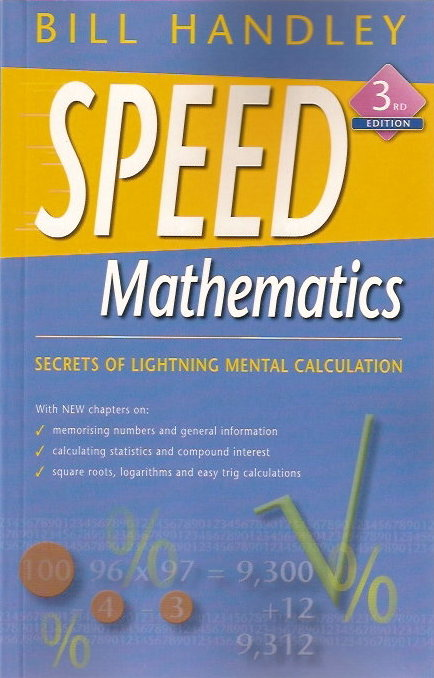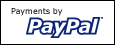# Get the brilliant new bookSpeed Mathematics 3rd edition

•Master basic tables in ten minutes
• Multiply large numbers mentally
• Learn easy methods for short and long division
• Learn easy methods to check your answers so you seem to never make a mistake
• Add and subtract fractions at a glance
• Square numbers and find square roots mentally
• Simple and easy checks for divisibility by any number
• Square feet and inches easily
• Fun method of division as easy as addition
• Easy introduction to logarithms
• Easy method for finding any root of any number
• Memorise numbers
• Explanation why the methods work
• Make maths fun

The book is written simply so anyone can understand. The book teaches simple strategies that will enable you to make lightning calculations—multiplication, division, addition and subtraction, squaring numbers or extracting square roots and cube roots. Add and subtract fractions at a glance. Solve compound interest problems easily, memorise numbers, learn how to use logarithms to make calculations easy. Solve right-angled triangles, calculate hypotenuse mentally. Improve your understanding of mathematics while you perform like a genius. People who excel at mathematics use better strategies than the rest of us; they are not necessarily more intelligent. You will learn these strategies. The methods make maths easy and fun.

This book is great for students, engineers, parents, teachers, in fact, anyone who enjoys working with numbers and anyone who is terrified of numbers. Home schoolers definitely need a copy of this inexpensive paperback. This new third edition has extra information and new chapters on memorising numbers, logarithms, calculating compound interest and right-angled triangles.

For AUD\$29.95, plus postage, (\$9 postage if ordered in Australia) this book is a great investment for your future and the future of your children.

Order online with PayPal